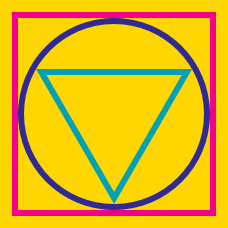Geometry

# Circumcircle of Triangle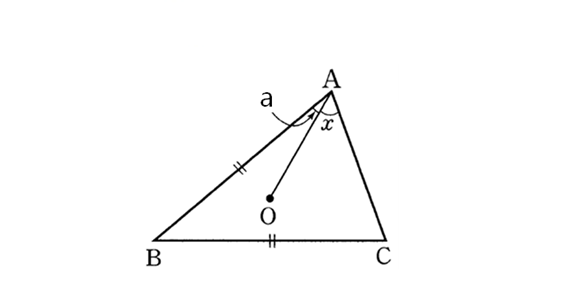In the above diagram, point $$O$$ is the circumcenter of $$\triangle ABC$$ and the lengths of $$\overline{AB}$$ and $$\overline{BC}$$ are the same, i.e. $$\lvert \overline{AB} \rvert=\lvert \overline{BC} \rvert.$$ If $$\angle OAB(=a)$$ is $$15^{\circ},$$ what is $$\angle OAC(=x)$$?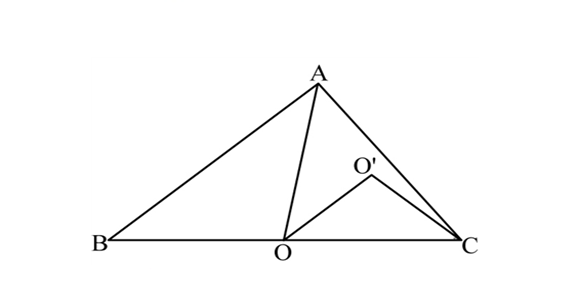In the above diagram, point $$O$$ is the circumcenter of $$\triangle ABC$$ and point $$O'$$ is the circumcenter of $$\triangle AOC.$$ If $$\angle ABO = 28^{\circ},$$ what is the value of $$\angle OO'C$$ in degrees?

Note: The above diagram is not drawn to scale.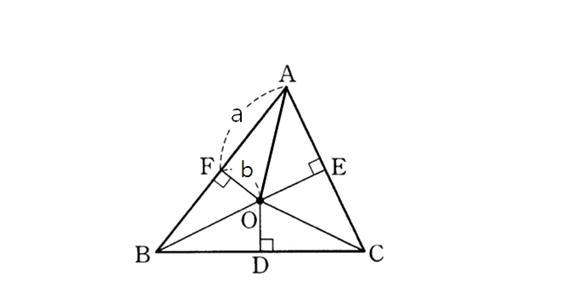In the above diagram, point $$O$$ is the circumcenter of $$\triangle ABC.$$ The length of $$\overline{AF}$$ is $$10(=a)$$ and the length of $$\overline{OF}$$ is $$3(=b).$$ If the area of $$\triangle ABC$$ is $$116,$$ what is the area of $$CDOE?$$

Note: The above diagram is not drawn to scale.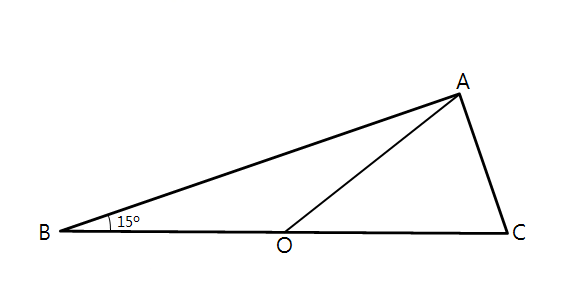In the above diagram, point $$O$$ is the circumcenter of $$\triangle ABC$$ and $$\angle ABC$$ is $$15^{\circ}.$$ If the radius of the circumscribed circle is $$16,$$ what is the area of $$\triangle ABC?$$

Note: The above diagram is not drawn to scale.In the above diagram, point $$O$$ is the circumcenter of $$\triangle ABC.$$ The length of $$\overline{AF}$$ is $$6(=a)$$ and the length of $$\overline{OF}$$ is $$5(=b).$$ If the area of $$\triangle ABC$$ is $$98,$$ what is the area of $$CDOE?$$

Note: The above diagram is not drawn to scale.

×